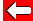# Lowest Common Denominators

Some common denominators are not very convenient due to their large size. It's a good idea to find the lowest common denominator (LCD).

The first check to make with all pairs of fractions is whether the smallest denominator is a factor of the other one.

e.g. 1/4 and 3/20 ... 4 is a factor of 20!

So, whilst we could multiply the denominators (and correct the numerators) to give 20/80 and 12/80 (which we could simplify), it's much easier to spot the factor (4) and alter only this fraction:

1/4 and 3/20 becomes 5/20 and 3/20.Go back a pageMaths MenuGo to next page